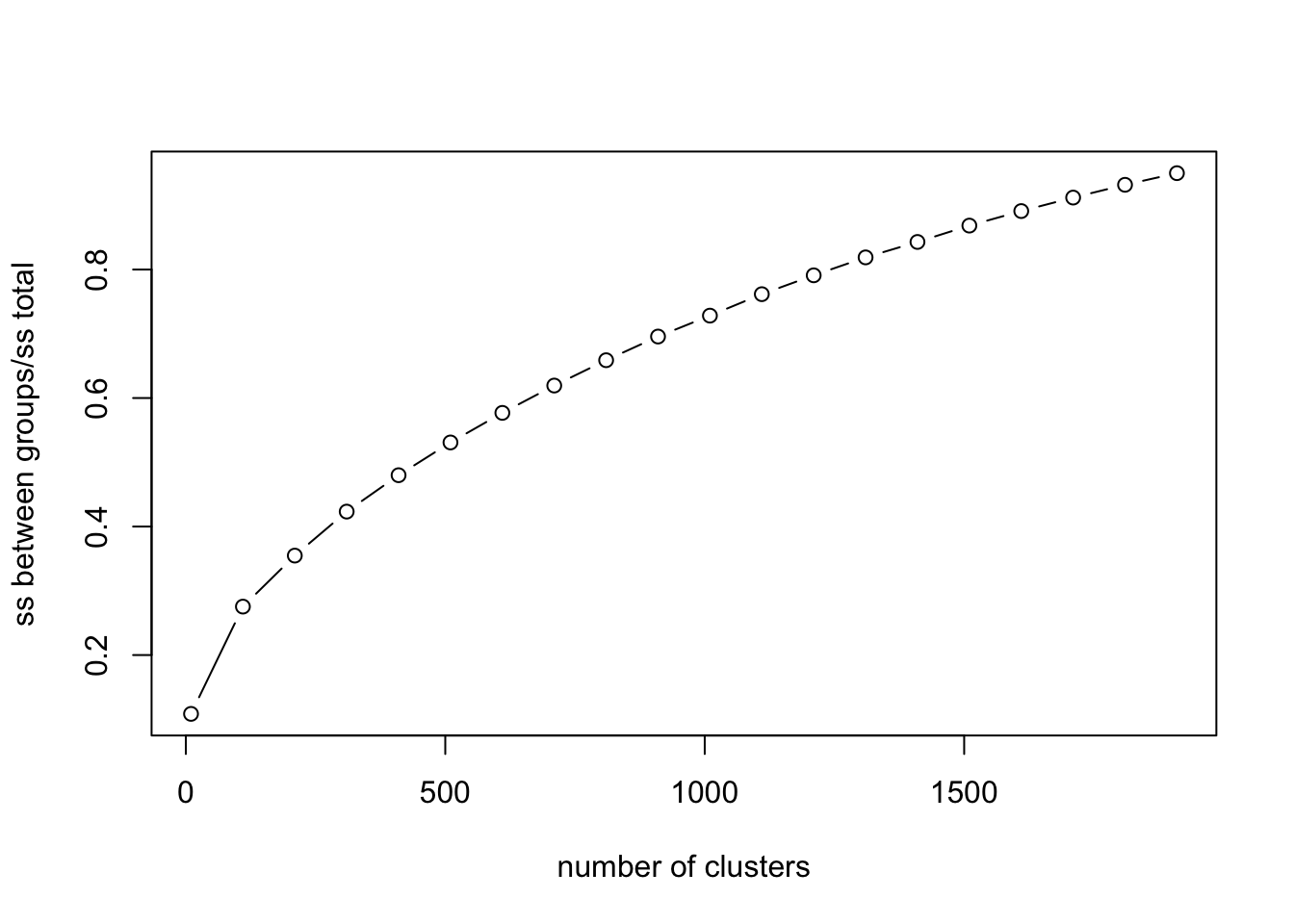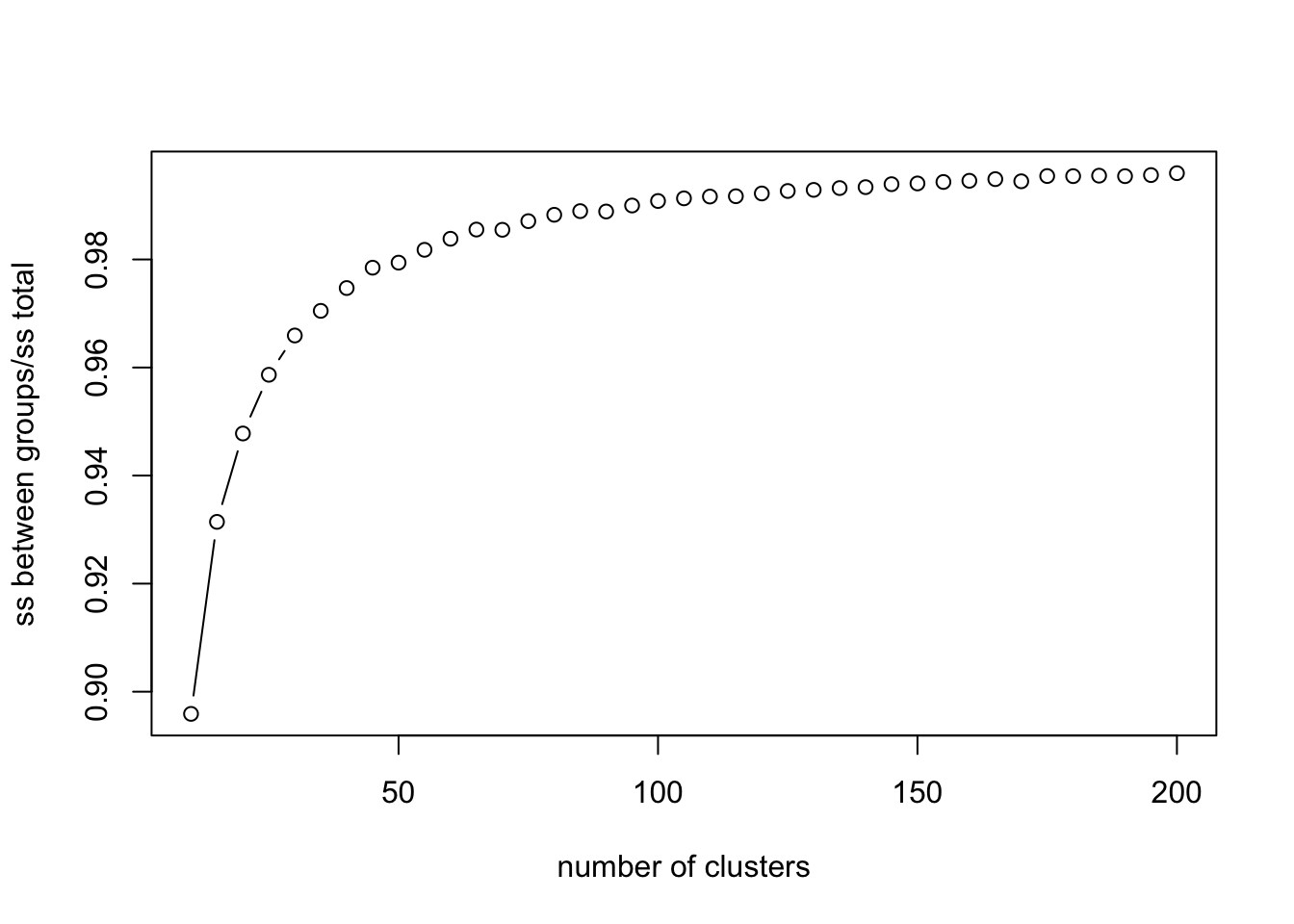# Overview

We have a few analyses that have been considered for write up that involve cluster analyses, namely using kmeans (stats::kmeans()). If we decide to cluster there is the issue of the number of clusters that should be specified given that kmeans() requires you to specify the number of groups in advance. There is some discussion about this in the machine learning literature given the flexible nature of the algorithm. We could come up wth a way of motivating the number of clusters selected given that, if we claim that the clustering in semantic space is psychologically real, the cluster membership plays into the analyses in an important way.

## A method for determining clusters

We can estimate the explanatory utility of group membership (different assignments to clusters) in terms of the relationship between variation in coordinate space between clusters against the total variation in coordinate space of words in multidimensional space. As the number of clusters increases towards the total number of words embedded in coordinate space, the between cluster sum of squares will naturally inch closer towards the sum of squares total. Ideally we find a number of clusters that differentiates itself from other cluster assignments in terms of this ratio. If we can’t do that, we could select a set of cluster assignments that doesn’t overfit the space but still represents the structure of words in the space in a reasonably small number of groups.

In the plots below the y-axis corresponds to the sum of squares between clusters divided by the sum of squares total.

### Clusters within Wikipedia semantic vectors

#### 300D space

We obtained semantic representations for all the gender-normed words in our corpus from Wikipedia generated from fastText (via Facebook). These representations lie in 300 dimensional continuous space. We can then apply kmeans across different values for k and plot the sum of squares between groups relative to total sum of squares. What we see is that it takes many clusters to approach a reasonable value for ss between groups/ss total. Also, the only elbow in the plot is early on, at a point in k where little variation is being explained.

wiki_vecs %>%
select(starts_with("V")) %>%
manipulate_kmeans(10, 2000, 100, 15)From there let’s take a specific value for k so that we can compare cluster membership to clusters derived from the already reduced t-SNE data described below. We can go with 50 given that 50 clusters in t-SNE derived coordinate space (below) explains about 98% of the total sum of squares.

wiki_50_clusters = kmeans(wiki_vecs[2:301], 50)
cluster_compare_df <- data.frame(cbind(wiki_vecs$word, wiki_50_clusters$cluster)) %>%
rename(word = X1, cluster_wiki_50 = X2) %>%
mutate(cluster_wiki_50 = as.numeric(cluster_wiki_50))

This cluster assignment (50 clusters) accounts for about 0.2060929 of the total variation in coordinate space.

#### 2D space

If we apply t-SNE to our 300D space and reduce the dimensionality down to 2 dimensions, we can follow the same procedure using kmeans. Below you can see variation accounted for on the y-axis, plotted as a function of increasing cluster assignment on the x-axis. Clusters much more easily account for variation in 2D space, with about 98% of variation accounted for by 50 clusters, and asymptote being reached not long after.

TSNE_DATA %>%
select(tsne_X, tsne_Y) %>%
manipulate_kmeans(10, 200, 5, 15)tsne_50_clusters = kmeans(TSNE_DATA[2:3], 50)
cluster_compare_df <- data.frame(cbind(TSNE_DATA$word, tsne_50_clusters$cluster)) %>%
rename(word = X1, cluster_tsne_50 = X2) %>%
mutate(cluster_tsne_50 = as.numeric(cluster_tsne_50)) %>%
right_join(cluster_compare_df)

Again selecting a value for k = 50, we can derive cluster membership for all the words in the set and join those cluster assignments with the assignments based on the 300D kmeans solution. If we do this we see that the correlation is r = 0.0782417, indicating that in a 50 cluster solution, the assignments in 300D and 2D aren’t correlated. In 300D the 50 cluster solution accounts for 0.2060929 of the overall variability in coordinate space, whereas in 2D, the 50 cluster solution accounts for 0.9808276 of the overall variability.